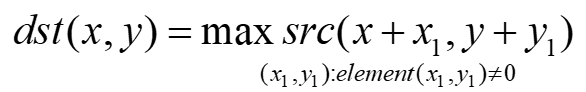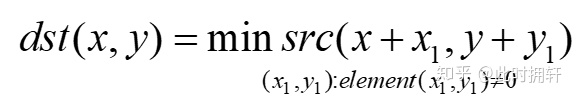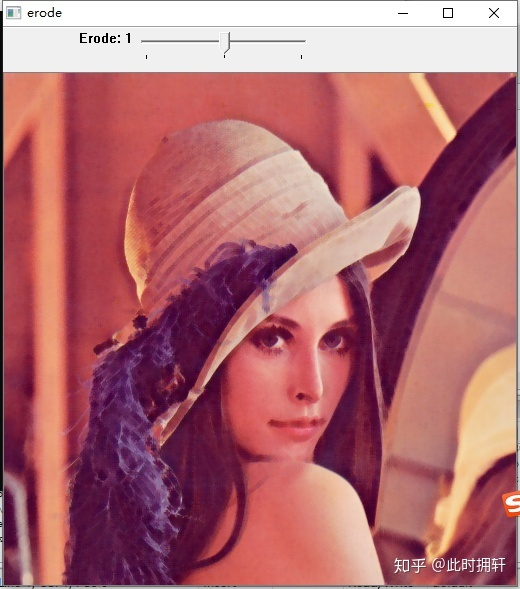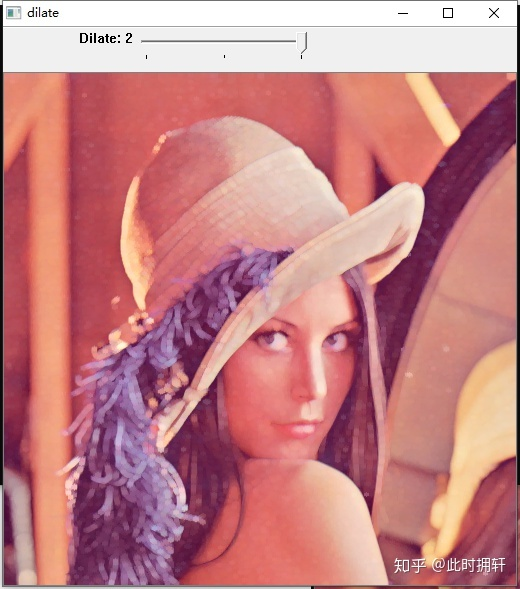# opencv 膨胀_形态学操作：腐蚀与膨胀

• 消除噪声；
• 分割出独立的图像元素，连接相邻的元素；
• 寻找图像中得明显的极大值区域或极小值区域；
• 求图像的梯度。void cv::dilate( InputArray src, OutputArray dst, InputArray kernel,
Point anchor = Point(-1,-1), int iterations = 1,
int borderType = BORDER_CONSTANT,
const Scalar& borderValue = morphologyDefaultBorderValue() );


void cv::erode( InputArray src, OutputArray dst, InputArray kernel,
Point anchor = Point(-1,-1), int iterations = 1,
int borderType = BORDER_CONSTANT,
const Scalar& borderValue = morphologyDefaultBorderValue() );


src : 输入图像，图像深度为CV_8U,CV_16U,CV_16S,CV_32F或CV_64F其中之一

dst : 输出的目标图像，和原图像有相同尺寸和大小

kernel : 腐蚀或者膨胀的卷积核，当为NULL时，表示的使用锚点位于中心的3x3的卷积核

anchor : 锚点位置，当为(-1,-1)时候，表示位于中心

iteration:表示迭代的腐蚀或者膨胀的次数

borderType ：用于推断图像外部像素的某种边界模式

borderValue：当边界为常数时的边界值

cv::Mat getStructuringElement(int shape, cv::Size ksize, cv::Point anchor = Point(-1,-1))


shape: 结构元素的形状，有以下三种：

MORPH_RECT ： 矩形

MORPH_CROSS : 交叉形状

MORPH_ELLIPSE: 椭圆形

ksize ：结构元素的大小

anchor : 指定锚点位置，当锚点为（-1，-1）表示锚点位于结构元素中心。

#include <iostream>
#include <opencv2/opencv.hpp>
cv::Mat srcImage;
cv::Mat srcImage1;
void OnErode(int pos,void *userdata)
{
cv::Mat dstImage;
cv::Mat element = cv::getStructuringElement(pos,cv::Size(5,5),cv::Point(-1,-1));
cv::erode(srcImage,dstImage,element);
cv::imshow("erode",dstImage);
}
void onDilate(int pos,void *userdata)
{
cv::Mat dstImage;
cv::Mat element = cv::getStructuringElement(pos,cv::Size(5,5),cv::Point(-1,-1));
cv::dilate(srcImage,dstImage,element);
cv::imshow("dilate",dstImage);
}
int main(int argc,char *argv[]) {
srcImage = cv::imread(argv);
if (!srcImage.data)
{
std::cerr << "fail to load image" << std::endl;
return -1;
}
srcImage1 = srcImage.clone();
cv::namedWindow("dilate",cv::WINDOW_AUTOSIZE);
cv::namedWindow("erode",cv::WINDOW_AUTOSIZE);
cv::createTrackbar("Dilate","dilate",0,2,onDilate);
cv::createTrackbar("Erode","erode",0,2,OnErode);
cv::imshow("dilate",srcImage);
cv::imshow("erode",srcImage1);
cv::waitKey(0);
cv::destroyAllWindows();
return 0;
}©️2020 CSDN 皮肤主题: 游动-白 设计师:白松林